# Mathematically modeling a real system in C#

• btb4198
I am trying to fit this:If those 20 points are not representative, why did you post them?I thought it was enough, I guess I was wrong . sorryHow many points do you need to do a Sinusoidal Curve fitting ?This guy :only used 12 points

#### btb4198

How can I mathematically model a real system and predict values from that system?

I have a real device that produces data that I can graph. The data looks like a sinewave.

I want to use an sinewave fit equation to predict some of the measurements because of how long it takes to run a measurement.

here is an example of some of the data I am seeing :

 1​ 65​ 2​ 63​ 3​ 62​ 4​ 63​ 5​ 65​ 6​ 66​ 7​ 67​ 8​ 68​ 9​ 69​ 10​ 70​ 11​ 71​ 12​ 72​ 13​ 73​ 14​ 74​ 15​ 75​ 16​ 77​ 17​ 78​ 18​ 79​ 19​ 80​ 20​ 81​

As you can see from the first 4 measurements, it does not produce a perfect sine wave

So,
A = 9.5
B = 71.5

Period = 2*π / w

w = 2*π/20 = π/10
but I am not sure since I think that 62 is because of noise ..
anyhow I get this when I graph it :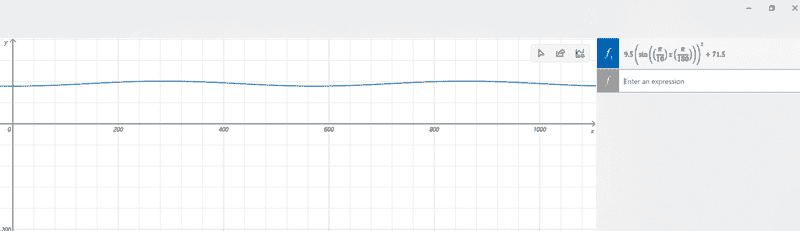which is not right..
Does anyone know an algorithm I can use or any free c# NuGet that can do this ?

Last edited:
Hi,
could you read back what you posted? It really looks nonsensical !

And: what are A and B ?

I Agree it would be useful for you to explicitly write out the model you are trying to fit.

Numerically this is probably pretty straightforward, any programming language (and even Microsoft Excel) is going to have a standardly available simple optimization library that will probably give you good results.

btb4198 said:
The data looks like a sinewave.

No, it looks like this: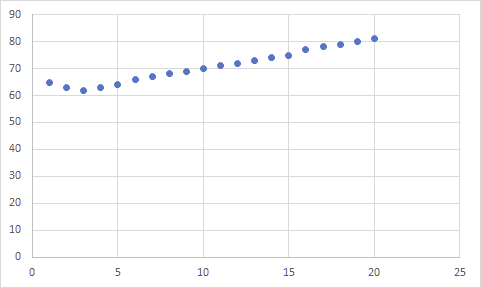BvU said:
Hi,
could you read back what you posted? It really looks nonsensical !

And: what are A and B ?

sorry about that , I just edited the post

No, it looks like this:
View attachment 290133
That was only with 20 points, I could do more points and it would look more like a sinusoidal wave but
that would be a lot of points and I did not know how much was needed for this post.

If those 20 points are not representative, why did you post them?

Office_Shredder said:
I Agree it would be useful for you to explicitly write out the model you are trying to fit.

Numerically this is probably pretty straightforward, any programming language (and even Microsoft Excel) is going to have a standardly available simple optimization library that will probably give you good results.
I am trying to fit this:
If those 20 points are not representative, why did you post them?
I thought it was enough, I guess I was wrong . sorry

Office_Shredder said:
I Agree it would be useful for you to explicitly write out the model you are trying to fit.

Numerically this is probably pretty straightforward, any programming language (and even Microsoft Excel) is going to have a standardly available simple optimization library that will probably give you good results.
I am trying to fit to this :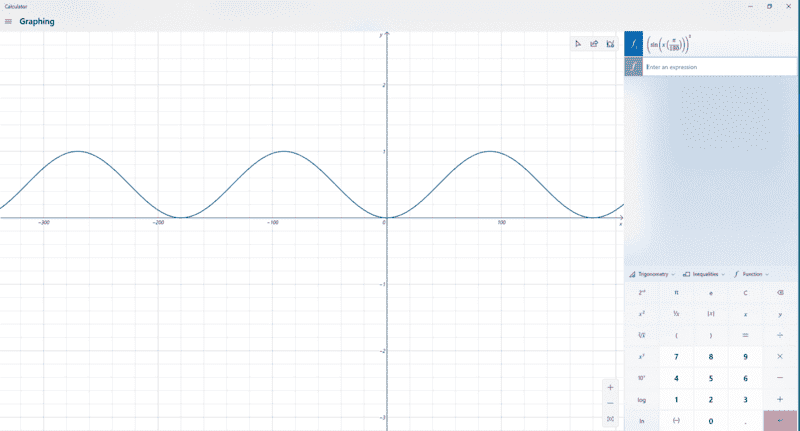Office_Shredder said:
I Agree it would be useful for you to explicitly write out the model you are trying to fit.

Numerically this is probably pretty straightforward, any programming language (and even Microsoft Excel) is going to have a standardly available simple optimization library that will probably give you good results.
I am trying to fit this:
BvU said:
Hi,
could you read back what you posted? It really looks nonsensical !

And: what are A and B ?

So
y = A sin(wX) + B

A is the amplitude of a wave
w is = to 2*π/ the period

is that not right ?

btb4198 said:
I am trying to fit this:

I thought it was enough, I guess I was wrong . sorry
How many points do you need to do a Sinusoidal Curve fitting ?
this guy :

only used 12 points

Office_Shredder said:
I Agree it would be useful for you to explicitly write out the model you are trying to fit.

Numerically this is probably pretty straightforward, any programming language (and even Microsoft Excel) is going to have a standardly available simple optimization library that will probably give you good results.
Sadly C# does not have come with a sinusoidal fit equation.
IDK why

All,
would more data points be useful ? again it take time to run it, hint why I wanted to use the sinusoidal fit.
I wanted to only take measurements around the low and high angles and then use the fit for the others.

btb4198 said:
Sadly C# does not have come with a sinusoidal fit equation.
IDK why
It also doesn't include a spreadsheet or a chess engine.

You know the functional form of what you are trying to fit. You can write a fitter yourself. If you can't do this, you can switch to a tool that does this. As @Office_Shredder points out, even Excel does this. If you Google "sinusoidal fitting excel" several sites have step by step instructions.

https://stackoverflow.com/questions/1211201/free-optimization-library-in-c-sharp

I can't promise the stuff linked there is the best/easiest to use but this is the kind of thing you are looking for. Generically there are libraries which expose a function called something like optim. Optim takes as arguments a function, and a starting guess for a solution (it will often have lots of optional arguments, ignore them for now). In this case your function is going to take a triple as its input: ##(A,B,\omega)## and return something like

$$\sum_{(x_i,y_i)}( A\sin(\omega x_i) + B - y_i)^2$$

Where ##(x_i,y_i)## are the initial data you have. Your goal is to find a value for ##(A,B,\omega)## which makes the output of this function as small as possible, that's what numerical optimization libraries do. The nice thing about this setup is it's very flexible - if you decide to add extra parameters or change the shape of yours function, you can just rerun it without doing any work.

It also doesn't include a spreadsheet or a chess engine.

You know the functional form of what you are trying to fit. You can write a fitter yourself. If you can't do this, you can switch to a tool that does this. As @Office_Shredder points out, even Excel does this. If you Google "sinusoidal fitting excel" several sites have step by step instructions.

If I can't graph it on a graphing calculator then I can't code it. lol That is why I am asking.
What am I doing wrong in my Fit equations?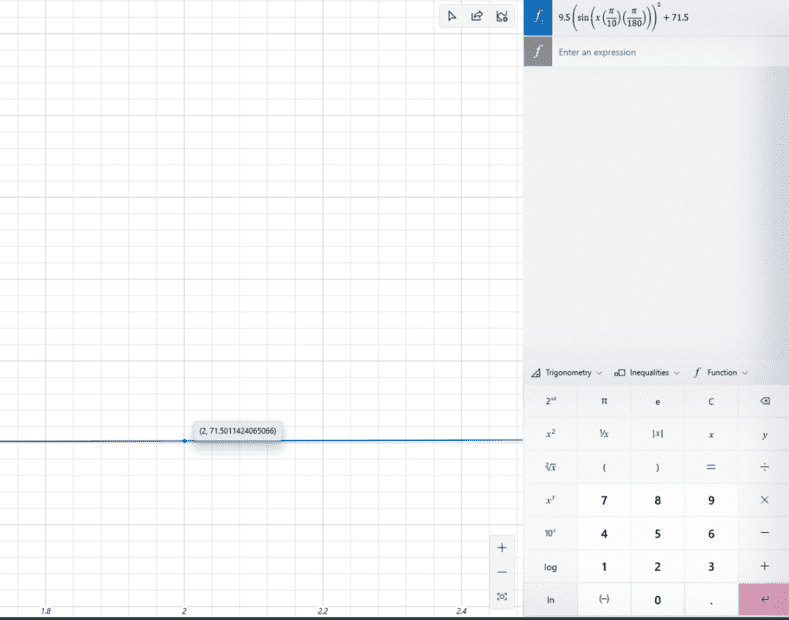if you look at the graph from my fit equation you will see that for angle 2 is say 71.50 but it should be 63. I do not even see 63 in the graph at all.
The lowest value I see is 71.5

Heaven only knows what you have done, so how can we tell you where you have gone wrong?

If you don't want to learn to code this in C#, but insist on C# as opposed to other tools people have suggested, I don't see a path forward.

Heaven only knows what you have done, so how can we tell you where you have gone wrong?

If you don't want to learn to code this in C#, but insist on C# as opposed to other tools people have suggested, I don't see a path forward.

wait I never said I did not want to learn how to code it myself. Only that I currently cannot do it by hand nor with a graphing calculator.

I can only code some thing, if I know how to do it .
I coded my own FFT but only after I learned how to do it by hand 1st.

Using the data from the tool, I solved for A B and w.

like in the video I posted above .

unfortunately the guy only show how to do that part by hand. then he start using a program to do the other part.
that is part I do not know how to do by hand.

IDK if I should have posted this in the math area or not.

but I feel like it is both .

Also, I have an entire program I wrote In c-sharp that control the tool. So I can't just switch to a new language.

So, how are things ?

btb4198 said:
That was only with 20 points, I could do more points and it would look more like a sinusoidal wave but
that would be a lot of points and I did not know how much was needed for this post.
Can you show a plot of the full data set ? We still don't see that
btb4198 said:
The data looks like a sinewave.

And:
Do you understand post #15 ?

btb4198 said:
If I can't graph it on a graphing calculator then I can't code it. lol That is why I am asking.
I fail to understand this kind of logic.
In addition it looks as if you can graph it. Just:
The vertical scale seems to be immense, so the fit looks like a horizontal line.

btb4198 said:
What am I doing wrong in my Fit equations?
Probably nothing (*). You will have to tell us exactly, step by step what you are doing and what the full input is before we can say anything sensible about that. Otherwise it will come down to telepathy and we are notoriously bad at that. lol.

And the rule "garbage in, garbage out" still holds up !

(*) Although: what is the meaning of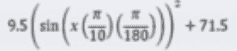btb4198 said:
The lowest value I see is 71.5
I wonder why you seem to be surprised about that##\ ##

Hi,

9.5 (Sin(x(π/10)(π/180)) ^2 + 7.15

So 9.5 is my A
you get A by Subtracting the biggest number from the smallest.

71.75 is my B You get B by adding the smallest number to the biggest number in the volume by two
I am multiplying my X by π/180 to converted from radians to degrees.

I am using sine squared to only get positive values.

w = π/10 because my My period is 20 different values

BvU said:
fail to understand this kind of logic.
In addition it looks as if you can graph it. Just:
The vertical scale seems to be immense, so the fit looks like a horizontal line.

My real life vertical scale is only 0 to 360 degrees, that is just how the computer Graphing calculator works.

BvU said:
And:
Do you understand post #15 ?
I think I do. I have not coded it yet. but I think I take my solved A, B ,and W values and keep lower them until I get the smallest sum.

I am not sure how to lower them .
is it just A-- B-- W--?

Do you lower A then B then W?

not sure.

if someone can do a handwritten example for me that would be the Best!
That is how I was able to code my FFT. I found a guy on Youtube that did it by hand on a whiteboard.

btb4198 said:
My period is 20 different values
Are you suggesting that the data we see graphed in post #4 is all you have and you assume it's periodical ? That's not a sine, it is a sawtooth !

btb4198 said:
I am multiplying my X by π/180
No you are not. You are multiplying it by (π/10) times (π/180)

btb4198 said:
to converted from radians to degrees
Huh ? Are you seriious ?

btb4198 said:
I am using sine squared to only get positive values.
There is no argument for that if you are fitting to ##A\sin \omega x + B##

btb4198 said:
9.5 (Sin(x(π/10)(π/180)) ^2 + 7.15

So 9.5 is my A
you get A by Subtracting the biggest number from the smallest.

71.75 is my B You get B by adding the smallest number to the biggest number in the volume by two
I am multiplying my X by π/180 to converted from radians to degrees.

I am using sine squared to only get positive values.

w = π/10 because my My period is 20 different values
Try to be more consistent in what you write. In post #1, you have B = 71.5. Above you have 7.15 in the first line, and 71.75 a few lines below.

The formula for your function could be simplified by first converting the input value from degrees to radians, and then doing the calculation in two steps using an intermediate value.
C:
// deg is the value in degrees; x is in radians
x = deg * Math.PI / 180;
intVal = Math.Sin( x * Math.PI / 10);
y = 9.5 * intVal * intVal + 71.5

btb4198 said:
My real life vertical scale is only 0 to 360 degrees, that is just how the computer Graphing calculator works.
This makes no sense to me. If you're working with any of the trig functions, one period would be either 0 to ##\pi## (tan and cotan) or 0 to ##2\pi## (sin, cos, and their reciprocals). In degrees these would be 0 to 180 degrees or 0 to 360 degrees. The outputs (along the vertical axis) of these functions are real numbers, not measures of angles. If you graph ##y = \sin(x)##, all of the output values will be between -1 and 1. If you graph ##y = 9.5 \sin^2(\frac{\pi x}{10}) + 71.5##, with x in radians, all of the outputs will lie in the interval 71.5 to 81.0. The resulting curve does not look like a sine wave, as there are no troughs as in actual sine waves.

Mark44 said:
Try to be more consistent in what you write. In post #1, you have B = 71.5. Above you have 7.15 in the first line, and 71.75 a few lines below.

The formula for your function could be simplified by first converting the input value from degrees to radians, and then doing the calculation in two steps using an intermediate value.
C:
// deg is the value in degrees; x is in radians
x = deg * Math.PI / 180;
intVal = Math.Sin( x * Math.PI / 10);
y = 9.5 * intVal * intVal + 71.5

This makes no sense to me. If you're working with any of the trig functions, one period would be either 0 to ##\pi## (tan and cotan) or 0 to ##2\pi## (sin, cos, and their reciprocals). In degrees these would be 0 to 180 degrees or 0 to 360 degrees. The outputs (along the vertical axis) of these functions are real numbers, not measures of angles. If you graph ##y = \sin(x)##, all of the output values will be between -1 and 1. If you graph ##y = 9.5 \sin^2(\frac{\pi x}{10}) + 71.5##, with x in radians, all of the outputs will lie in the interval 71.5 to 81.0. The resulting curve does not look like a sine wave, as there are no troughs as in actual sine waves.
Mark44,

Sorry but I think I might have missed understood what period means from the YouTube video I watched.
I was under the impression it was your start position - end position / sampling rate.
So I start my motor at angle -10 and move it to angle 10 at a sampling rate of 1.
I take a reading from my CCD array on every angle.
The motor has the ability to rotate from 0 - 360 at a smallest step of 0.02 degrees.
but that would take way to long.
So I only did 20 points around the bottom if the output data.
ok so at angles 0, 180 , and 360 the CCD Array ideally should read a transmission of 0% but really I see a value around 4%.
note 0 and 360 is the same location because the motor rotates in a circle.
and at angles 90, 270, ideally I should see a value of 80%
anyhow, I am wrong about the period?

btb4198 said:
Sorry but I think I might have missed understood what period means from the YouTube video I watched.
I was under the impression it was your start position - end position / sampling rate.
I don't see that sampling rate has anything to do with the period. A periodic function f is one for which ##f(x + p) = f(x)##, where p is the period. IOW, the period is the smallest interval in which a complete cycle of the function occurs. For the sine function (##y = \sin(x)##), x is typically in real number units; i.e., radians, and the period is ##2\pi##. In degrees that is 360.

Here are some basic ideas about transformations of functions.
##y = \sin(x)## - period is ##2\pi##, range is [-1, 1]
##y = \sin( \omega x)## - period is ##\frac {2\pi} \omega##, range is [-1, 1]
##y = A\sin( \omega x)## - period is ##\frac {2\pi} \omega##, range is [-A, A]
##y = \sin^2(x)## - period is ##\pi##, range is [0, 1]
##y = A\sin^2(x)## - period is ##\pi##, range is [0, A]
##y = A\sin^2(\omega x)## - period is ##\frac \pi \omega##, range is [0, A]
##y = A\sin^2(\omega x) + B## - period is ##\frac \pi \omega##, range is [B, B + A]

•feynman_fan and berkeman
There is an old program named 'Sieclator' that will do a polynomial fit to data points you enter. Sieclator uses a curve-fit plug-in called 'PForm1Setup.exe'. Several Sieclator download sites can be found with:

Sieclator was part of a software package called 'Revelator' which seems to have disappeared. Here is the author's note from 2009:
url: http://users.bigpond.net.au/Revelator
Email: revelator.programs@hotmail.com

Please note:- This website will close soon.

On September 30, 2009 the web host used to distribute Revelator programs will cease to exist.

Revelator programs will remain available to about the time BigPond pulls its web plug. Web hosts can be transient; a problem always is to locate a reliable one. Until such time, Revelator programs will be unavailable after the above date, though the above Email address will survive: good old hotmail.com.

(R.F. La Fontaine)

Cheers,
Tom

p.s. Some high end handheld calculators at least used to have curvefit capability.

Last edited:
A polynomial fit is useless for a periodic function (and although it was slow in coming the OP's latest description of the source of the data indicates a periodic solution with a known period).

Mark44 said:
I don't see that sampling rate has anything to do with the period. A periodic function f is one for which ##f(x + p) = f(x)##, where p is the period. IOW, the period is the smallest interval in which a complete cycle of the function occurs. For the sine function (##y = \sin(x)##), x is typically in real number units; i.e., radians, and the period is ##2\pi##. In degrees that is 360.

Here are some basic ideas about transformations of functions.
##y = \sin(x)## - period is ##2\pi##, range is [-1, 1]
##y = \sin( \omega x)## - period is ##\frac {2\pi} \omega##, range is [-1, 1]
##y = A\sin( \omega x)## - period is ##\frac {2\pi} \omega##, range is [-A, A]
##y = \sin^2(x)## - period is ##\pi##, range is [0, 1]
##y = A\sin^2(x)## - period is ##\pi##, range is [0, A]
##y = A\sin^2(\omega x)## - period is ##\frac \pi \omega##, range is [0, A]
##y = A\sin^2(\omega x) + B## - period is ##\frac \pi \omega##, range is [B, B + A]
OH! right I remember that from school now. Sorry I think that YouTube video confused me.

so ##y = A\sin^2(x)## - period is ##\pi##, range is [0, A]

because my signal repeats every 180 180 degrees and that is π on a unit circle cool I understand that now.

So w = 2 because 2 π / π = 2

so now I have this: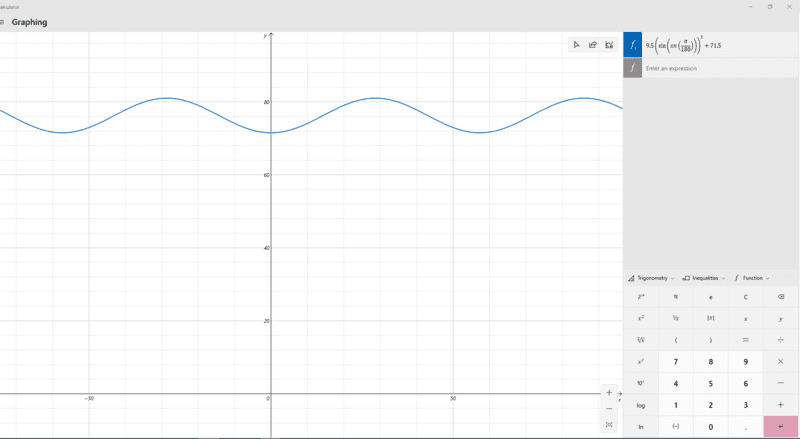pbuk said:
A polynomial fit is useless for a periodic function (and although it was slow in coming the OP's latest description of the source of the data indicates a periodic solution with a known period).
Sorry, I just didn't know what information was useful or not.

All I was able to get everything to work !
I can now graph a working Fit equation from my data by hand.
Thanks everyone that helped me

Can we see something of the results ?

BvU said:
Can we see something of the results ?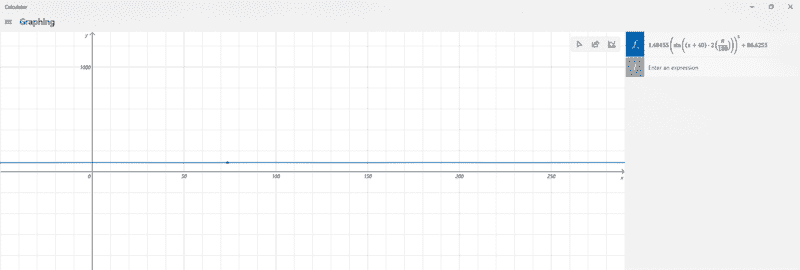This is a fit that I did today. And it fit the data that I measure. it is off at little as you do down. and I think it is because I did not iterate it. I think to iterated it , I would have to solute for A B and w again . I just do not know when to stop.

Last edited:
•pbuk
btb4198 said:
View attachment 290391

This is a fit that I did today. And it fit the data that I measure. it is off at little as you do down. and I think it is because I did not iterate it. I think to iterated it , I would have to solute for A B and w again . I just do not know when to stop.
You should adjust the vertical scale on your graph. What you show here looks like nothing more than a horizontal line, ##y \approx 100##.

Mark44 said:
You should adjust the vertical scale on your graph. What you show here looks like nothing more than a horizontal line, ##y \approx 100##.
I have been trying... but the graphing calculator does not let me... it is very frustrating, I am only using it because it comes with my computer .

btb4198 said:
I have been trying... but the graphing calculator does not let me... it is very frustrating, I am only using it because it comes with my computer .